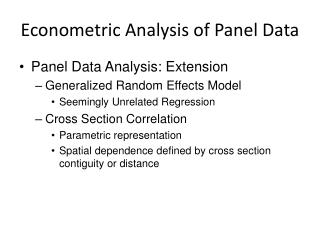DownloadDownload PresentationEconometric Analysis of Panel Data

# Econometric Analysis of Panel Data

Télécharger la présentation## Econometric Analysis of Panel Data

- - - - - - - - - - - - - - - - - - - - - - - - - - - E N D - - - - - - - - - - - - - - - - - - - - - - - - - - -
##### Presentation Transcript

1. Econometric Analysis of Panel Data • Panel Data Analysis: Extension • Generalized Random Effects Model • Seemingly Unrelated Regression • Cross Section Correlation • Parametric representation • Spatial dependence defined by cross section contiguity or distance

2. Panel Data Analysis: Extension Generalized Random Effects Model

3. Panel Data Analysis : Extension Seemingly Unrelated Regression

4. Panel Data Analysis : Extension • Cross Section Correlation • Unobserved heterogeneity: fixed effects or random effects • OLS with robust inference • GLS allowing time serial correlation

5. Panel Data Analysis : Extension • Cross Section Correlation • Parametric Representation

6. Panel Data Analysis : Extension Spatial Lag Variables Spatial Weights

7. Panel Data Analysis : Extension • Spatial Lag Model • OLS is biased and inconsistent • Unobserved heterogeneity: fixed effects or random effects • Observed heterogeneity

8. Panel Data Analysis : Extension • Spatial Error Model • Unobserved heterogeneity • Fixed effects • Random effects • Observed heterogeneity

9. Panel Data Analysis : Extension • Spatial Panel Data Analysis • Model specification could be a mixed structure of spatial lag and spatial error model. • Unobserved heterogeneity could be fixed effects or random effects. • OLS is biased and inconsistent; Consistent IV or 2SLS should be used, with robust inference. • If normality assumption of the model is maintained, efficient ML estimation could be used but with computational complexity. • Efficient GMM estimation is recommended.

10. Panel Data Analysis : Extension • Panel Spatial Model Estimation • IV / 2SLS / GMM • Instrumental variables for the spatial lag variable Wyt: [Xt, WXt, W2Xt,…] • W is a predetermined spatial weights matrix based on geographical contiguity or distance:

11. Panel Data Analysis : Extension Space-Time Dynamic Model Arellano-Bond estimator may be extended to include cross section correlation in the space-time dynamic models.

12. Example: U. S. Productivity • The Model (Munnell ) • One-way panel data model • 48 U.S. lower states • 17 years from 1970 to 1986 • Variables: gsp (gross state output); cap (private capital); 3 components of public capital (hwy, water, util); emp (labor employment); unemp (unemployment rate)

13. Example: U. S. Productivity • Spatial Panel Data Model • Cross Section Correlation • Cross section dependence is defined by state contiguity: if state i is adjacent with state j, then wij=1; otherwise wij=0. The spatial weights matrix W is then row-standardized with diagonal 0. • Pooled, fixed effects, random effects models are all biased and inconsistent. IV or 2SLS methods should be used with proper instruments.

14. Example: U. S. Productivity • Spatial Panel Data Model • Space-Time Dynamics